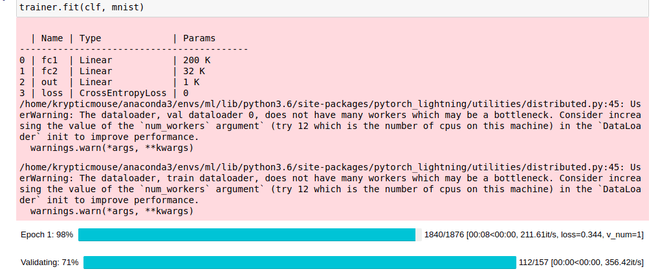GeeksforGeeks App
Open AppBrowser
Continue

# Understanding PyTorch Lightning DataModules

PyTorch Lightning aims to make PyTorch code more structured and readable and that not just limited to the PyTorch Model but also the data itself. In PyTorch we use DataLoaders to train or test our model. While we can use DataLoaders in PyTorch Lightning to train the model too, PyTorch Lightning also provides us with a better approach called DataModules. DataModule is a reusable and shareable class that encapsulates the DataLoaders along with the steps required to process data. Creating dataloaders can get messy that’s why it’s better to club the dataset in the form of DataModule. Its recommended that you know how to define a neural network using PyTorch Lightning.

Installing PyTorch Lightning:

Installing Lightning is the same as that of any other library in python.

`pip install pytorch-lightning`

Or if you want to install it in a conda environment you can use the following command:-

`conda install -c conda-forge pytorch-lightning`

## Pytorch Lightning DataModule Format

To define a Lightning DataModule we follow the following format:-

```import pytorch-lightning as pl

class DataModuleClass(pl.LightningDataModule):
def __init__(self):
#Define required parameters here

def prepare_data(self):
# Define steps that should be done
# on only one GPU, like getting data.

def setup(self, stage=None):
# Define steps that should be done on
# every GPU, like splitting data, applying
# transform etc.

# Return DataLoader for Training Data here

# Return DataLoader for Validation Data here

# Return DataLoader for Testing Data here```

Note: The names of the above functions should be exactly the same.

## Understanding the DataModule Class

For this article, I’ll be using MNIST data as an example. As we can see, the first requirement to create a Lightning DataModule is to inherit the LightningDataModule class in pytorch-lightning:

```import pytorch-lightning as pl

class DataModuleMNIST(pl.LightningDataModule):```

### __init__() method:

It is used to store information regarding batch size, transforms, etc.

```def __init__(self):
super().__init__()
self.batch_size = 32
self.transform = transforms.Compose([
transforms.ToTensor()
])```

### prepare_data() method:

This method is used to define the processes that are meant to be performed by only one GPU. It’s usually used to handle the task of downloading the data.

```def prepare_data(self):

### setup() method:

This method is used to define the process that is meant to be performed by all the available GPU. It’s usually used to handle the task of loading the data.

```def setup(self, stage=None):
train=True, transform=self.transform)

self.train_data, self.valid_data = random_split(data, [55000, 5000])

train=False, transform=self.transform)```

This method is used to create a training data dataloader. In this function, you usually just return the dataloader of training data.

```def train_dataloader(self):

This method is used to create a validation data dataloader. In this function, you usually just return the dataloader of validation data.

```def val_dataloader(self):

This method is used to create a testing data dataloader. In this function, you usually just return the dataloader of testing data.

```def test_dataloader(self):

### Training Pytorch Lightning Model Using DataModule:

In Pytorch Lighting, we use Trainer() to train our model and in this, we can pass the data as DataLoader or DataModule. Let’s use the model I defined in this article here as an example:

```class model(pl.LightningModule):
def __init__(self):
super(model, self).__init__()
self.fc1 = nn.Linear(28*28, 256)
self.fc2 = nn.Linear(256, 128)
self.out = nn.Linear(128, 10)
self.lr = 0.01
self.loss = nn.CrossEntropyLoss()

def forward(self, x):
batch_size, _, _, _ = x.size()
x = x.view(batch_size, -1)
x = F.relu(self.fc1(x))
x = F.relu(self.fc2(x))
return self.out(x)

def configure_optimizers(self):

def training_step(self, train_batch, batch_idx):
x, y = train_batch
logits = self.forward(x)
loss = self.loss(logits, y)
return loss

def validation_step(self, valid_batch, batch_idx):
x, y = valid_batch
logits = self.forward(x)
loss = self.loss(logits, y)```

Now to train this model we’ll create a Trainer() object and fit() it by passing our model and datamodules as parameters.

```clf = model()
mnist = DataModuleMNIST()
trainer = pl.Trainer(gpus=1)
trainer.fit(clf, mnist)```

Below the full implementation:

## Python3

 `# import module``import` `torch `` ` `# To get the layers and losses for our model``from` `torch ``import` `nn ``import` `pytorch_lightning as pl `` ` `# To get the activation function for our model``import` `torch.nn.functional as F `` ` `# To get MNIST data and transforms``from` `torchvision ``import` `datasets, transforms`` ` `# To get the optimizer for our model``from` `torch.optim ``import` `SGD `` ` `# To get random_split to split training``# data into training and validation data``# and DataLoader to create dataloaders for train, ``# valid and test data to be returned``# by our data module``from` `torch.utils.data ``import` `random_split, DataLoader `` ` `class` `model(pl.LightningModule): ``    ``def` `__init__(``self``): ``        ``super``(model, ``self``).__init__() ``         ` `        ``# Defining our model architecture``        ``self``.fc1 ``=` `nn.Linear(``28``*``28``, ``256``) ``        ``self``.fc2 ``=` `nn.Linear(``256``, ``128``) ``        ``self``.out ``=` `nn.Linear(``128``, ``10``) ``         ` `        ``# Defining learning rate``        ``self``.lr ``=` `0.01``         ` `        ``# Defining loss ``        ``self``.loss ``=` `nn.CrossEntropyLoss() ``   ` `    ``def` `forward(``self``, x):``       ` `          ``# Defining the forward pass of the model``        ``batch_size, _, _, _ ``=` `x.size() ``        ``x ``=` `x.view(batch_size, ``-``1``) ``        ``x ``=` `F.relu(``self``.fc1(x)) ``        ``x ``=` `F.relu(``self``.fc2(x)) ``        ``return` `self``.out(x) ``   ` `    ``def` `configure_optimizers(``self``):``       ` `          ``# Defining and returning the optimizer for our model``        ``# with the defines parameters``        ``return` `torch.optim.SGD(``self``.parameters(), lr ``=` `self``.lr) ``   ` `    ``def` `training_step(``self``, train_batch, batch_idx): ``       ` `          ``# Defining training steps for our model``        ``x, y ``=` `train_batch ``        ``logits ``=` `self``.forward(x) ``        ``loss ``=` `self``.loss(logits, y) ``        ``return` `loss ``   ` `    ``def` `validation_step(``self``, valid_batch, batch_idx): ``       ` `        ``# Defining validation steps for our model``        ``x, y ``=` `valid_batch ``        ``logits ``=` `self``.forward(x) ``        ``loss ``=` `self``.loss(logits, y)`` ` `class` `DataModuleMNIST(pl.LightningDataModule):``    ``def` `__init__(``self``):``        ``super``().__init__()``         ` `        ``# Directory to store MNIST Data``        ``self``.download_dir ``=` `''``         ` `        ``# Defining batch size of our data``        ``self``.batch_size ``=` `32``         ` `        ``# Defining transforms to be applied on the data``        ``self``.transform ``=` `transforms.Compose([``            ``transforms.ToTensor()``        ``])`` ` `    ``def` `prepare_data(``self``):``       ` `          ``# Downloading our data``        ``datasets.MNIST(``self``.download_dir, ``                       ``train ``=` `True``, download ``=` `True``)``         ` `        ``datasets.MNIST(``self``.download_dir,``                       ``train ``=` `False``, download ``=` `True``)`` ` `    ``def` `setup(``self``, stage``=``None``):``       ` `          ``# Loading our data after applying the transforms``        ``data ``=` `datasets.MNIST(``self``.download_dir,``                              ``train ``=` `True``, ``                              ``transform ``=` `self``.transform)``         ` `        ``self``.train_data, ``self``.valid_data ``=` `random_split(data,``                                                        ``[``55000``, ``5000``])`` ` `        ``self``.test_data ``=` `datasets.MNIST(``self``.download_dir,``                                        ``train ``=` `False``,``                                        ``transform ``=` `self``.transform)`` ` `    ``def` `train_dataloader(``self``):``       ` `          ``# Generating train_dataloader``        ``return` `DataLoader(``self``.train_data, ``                          ``batch_size ``=` `self``.batch_size)`` ` `    ``def` `val_dataloader(``self``):``       ` `          ``# Generating val_dataloader``        ``return` `DataLoader(``self``.valid_data,``                          ``batch_size ``=` `self``.batch_size)`` ` `    ``def` `test_dataloader(``self``):``       ` `        ``# Generating test_dataloader``        ``return` `DataLoader(``self``.test_data,``                          ``batch_size ``=` `self``.batch_size)`` ` `clf ``=` `model() ``mnist ``=` `DataModuleMNIST() ``trainer ``=` `pl.Trainer()``trainer.fit(clf, mnist) `

Output:My Personal Notes arrow_drop_up